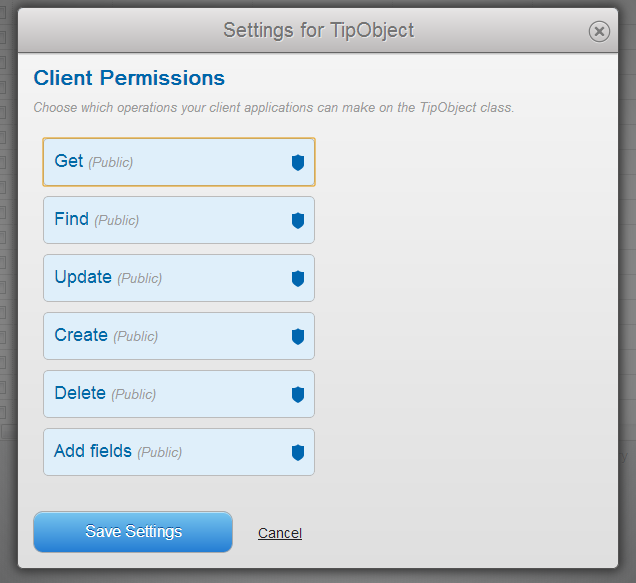Thanks for this find comes to me from Victor Yelizarov. He reported to me an odd issue with sorting and query of queries. Consider the following code sample:

`<cfset q = queryNew("number","Decimal")>`

`<cfset queryAddRow(q,5)> <cfset q["number"] = 10.1> <cfset q["number"] = 0.01> <cfset q["number"] = 22.75> <cfset q["number"] = 21.05> <cfset q["number"] = 8.33>`

`<cfquery name="q" dbtype="query"> SELECT number FROM q ORDER BY number DESC </cfquery>`

`<cfdump var="#q#">`

We know that ColdFusion looks at the query metadata to figure out how to do sorting. In this case he explicitly set the column type to decimal. That should have been enough. But from the result you can see otherwise...So obviously this is a bug - how do we get around it? If you JavaCast when setting the values, that works. If you multiply the values by one, that also works. But an easier solution, and one that may be useful to remember anyway, is to cast in your query of query.

`<cfquery name="q3" dbtype="query"> SELECT cast(number as decimal) as n FROM q ORDER BY n DESC </cfquery>`